﻿ 运动物体引力场传播的相对性原理

# 运动物体引力场传播的相对性原理Relativity Principle of Gravitational Field Propagation of Moving Objects

Abstract: Whether gravitational field is transmitted by gravitons or by gravitational waves that cause the curvature of space-time, modern physics has not found the necessary medium for the propagation of gravitational field. The magnitude and direction of the velocity of gravitational field’ propagation are stable in space with gravitational field spreading around from the position where generated. All these characteristics lead to the discoveries of the reference system closest to absolute rest and the relativity principle of the gravitational field of moving objects. The gravitational field intensity around a moving object will change comparing with that around the object in static state. The corresponding gravitational field intensity in front of the moving object will become weaker and the surfaces of the equal field intensity will become denser, while behind, the opposite. The object in front of the moving object will feel farther away from the moving object than their actual distance, while feeling less away when behind. The position of a moving object perceived by other objects lags behind the actual position. When only considering the gravitational field of the sun with other factors ignored, it can be deduced that the earth’s orbit around the sun is actually circular with the sun not in the center of the orbit which lags behind the sun by a certain distance. Based on the data measured by scientists so far, it can be calculated that the Milky Way with the solar system moves at a speed of up to 5000 km/s in space.

1. 引言

《量子力学》  认为引力是通过一种没有质量、永远相吸的粒子——引力子来传递的，引力子的传播速度是光速。《相对论》  认为引力是有质量的物体导致时空弯曲的结果，质量的改变或物体位置的改变会以引力波的形式向外辐射，从而把时空弯曲的改变以光速传播开来。2012年12月，中国科学院地质与地球物理研究所汤克云研究员领衔、中国地震局和中国科学院大学有关科研人员组成的科学团组在实施对日食期间的固体潮观测后，成功获得“引力场以光速传播”的观测证据，从而较准确地间接证明了引力场以光速传播。汤克云教授在《零速近似的牛顿引力公式掩盖了引力以光速传播的本质》  里进行了很好的阐述。

2. 引力场在空间的传播特点及静止参照系的建立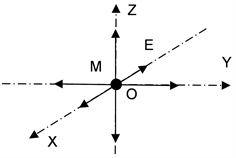Figure 1. The gravitational field propagates uniformly in space around M at a fixed speed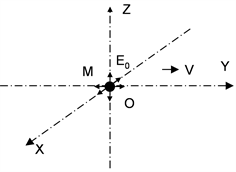Figure 2. Gravitational field E0 generated by M at point O at t0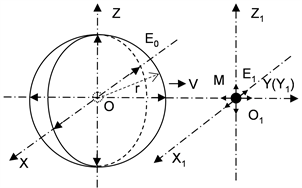Figure 3. The positions of gravitational field E0 and E1 generated by M at point O and O1 at t1

3. 运动物体引力场传播的相对性原理

3.1. 静止物体的引力场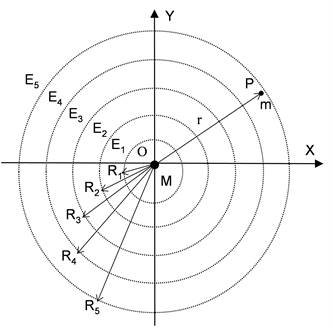Figure 4. Curves of equal gravitational field intensity of static M in X-Y plane

${f}_{j}=G\frac{Mm}{{r}^{2}}$，G为万有引力常数 (1)

P点处物体M的引力场强为：

${E}_{j}=\frac{{f}_{j}}{m}=G\frac{M}{{r}^{2}}$ (2)

3.2. 运动物体的引力场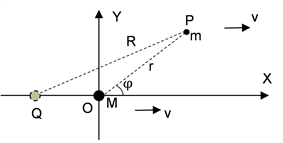Figure 5. Calculation of gravitational field of moving M

$\frac{QO}{v}=\frac{QP}{s}$，s为引力场传播速度 (3)

$QO=\frac{QP}{s}v=\frac{v}{s}R$ (4)

$Q{P}^{2}=Q{O}^{2}+O{P}^{2}-2QO\cdot OP\cdot \mathrm{cos}\left(\pi -\phi \right)$ (5)

${R}^{2}={\left(\frac{v}{s}R\right)}^{2}+{r}^{2}+2\frac{v}{s}R\cdot r\cdot \mathrm{cos}\phi$ (6)

$R=\frac{svr\mathrm{cos}\phi ±sr\sqrt{{s}^{2}-{v}^{2}{\mathrm{sin}}^{2}\phi }}{{s}^{2}-{v}^{2}}=\frac{sv\mathrm{cos}\phi ±s\sqrt{{s}^{2}-{v}^{2}{\mathrm{sin}}^{2}\phi }}{{s}^{2}-{v}^{2}}r$ (7)

$R=kr=\frac{sv\mathrm{cos}\phi +s\sqrt{{s}^{2}-{v}^{2}{\mathrm{sin}}^{2}\phi }}{{s}^{2}-{v}^{2}}r$ (8)

${f}_{d}=G\frac{Mm}{{R}^{2}}=G\frac{Mm}{{\left(kr\right)}^{2}}=\frac{1}{{k}^{2}}{f}_{j}$

P点的引力场强为

${E}_{d}=\frac{{f}_{d}}{m}=G\frac{M}{{R}^{2}}=G\frac{M}{{\left(kr\right)}^{2}}=\frac{1}{{k}^{2}}{E}_{j}$ (9)

$\mathrm{cos}\phi =-\frac{v}{2s}$ 时，k = 1，k−2 = 1，R = r。假设 ${\phi }_{1}=\mathrm{arccos}\left(-\frac{v}{2s}\right)$，由于 $v\ll s$，φ1略大于 $\frac{\pi }{2}$，如图6所示。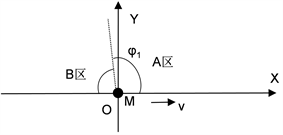Figure 6. The variation boundary of the gravitational field of moving M

$0\le \phi <{\phi }_{1}$ 时，k > 1，k−2 > 1，R > r，这意味着m位于图6中A区时感受的M的引力场强比二者处于静止状态时小，m感觉到的与M的距离R比实际距离r长。

${\phi }_{1}<\phi \le \pi$ 时，k < 1，k−2 < 1，R < r，这意味着m位于图6中B区时感受的M的引力场强比二者处于静止状态时要大，m感觉到的与M的距离R比实际距离r短。

$R=\frac{sv\mathrm{cos}\phi +s\sqrt{{s}^{2}-{v}^{2}{\mathrm{sin}}^{2}\phi }}{{s}^{2}-{v}^{2}}r$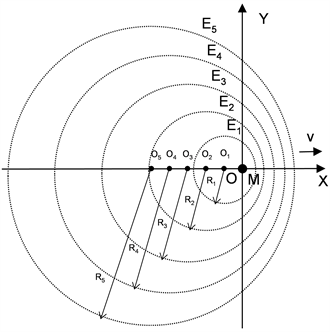Figure 7. Curves of equal gravitational field intensity of moving M in the X-Y plane

· 物体M相对静止参照系运动时，和M静止时相比其引力场强将产生变化。 如果忽略图6所示φ1与π/2差值的影响，则M运动前方相应位置的引力场将变弱，对应等场强面变密；M运动后方的相应位置引力场将变强，对应等场强面变疏。所有引力场的产生位置都滞后M实际的位置；

· 物体M相对静止参照系运动速度越大，物体周围相应位置的引力场强变化越大，变化系数为k−2

· 物体M相对静止参照系运动时，如果忽略图6所示φ1与π/2差值的与影响，位于M运动前方且和M相对位置保持不变的物体m感觉到的与M的距离比实际距离要长，而位于M运动后方且和M相对位置保持不变的物体m感觉到的与M的距离比实际距离要短，变化系数均为k。物体m感觉到的M的位置滞后M的实际位置。

4. 物体在运动物体引力场中的受力及运动特点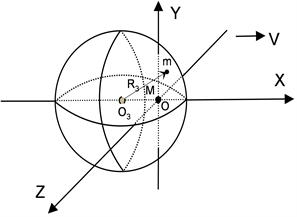Figure 8. In 3-D space m moves on the surface of ball O3 in the gravitational field of moving M

· 物体M相对静止坐标系静止时，其引力场中的物体m将有可能围绕物体M做圆周运动，M对m的感应点就是M的确在点；

· 物体M相对静止坐标系运动时，其引力场中的物体m将有可能围绕物体M对m的感应点做圆周运动。 M对m的感应点滞后M的确在点，m运行轨迹半径不变时，M速度越大，滞后距离越大。

5. 地球在相对静止参照系运动的太阳的引力场中的受力及运动分析Figure 9. The earth moves in a circle around the induction point G in the gravitational field of the sun

$R=kr=\frac{sv\mathrm{cos}\phi +s\sqrt{{s}^{2}-{v}^{2}{\mathrm{sin}}^{2}\phi }}{{s}^{2}-{v}^{2}}r$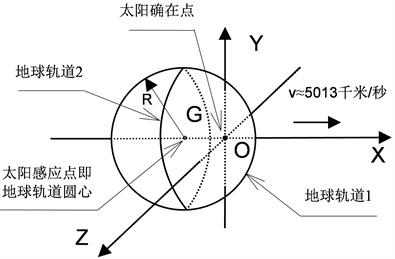Figure 10. The change of the earth's orbit around the sun

6. 太阳系各行星近日点分析

$R=\frac{sv\mathrm{cos}\phi +s\sqrt{{s}^{2}-{v}^{2}{\mathrm{sin}}^{2}\phi }}{{s}^{2}-{v}^{2}}r$

φ = 0时可求得太阳系各大行星近日点的计算值r，如表1所示。Table 1. Comparison between calculated and actual values of planetary perihelion (km)

· 由于v = 5013千米/秒是第5节中以地球实际近日点值计算得到的，所以上表中地球近日点的计算值与实际值的误差为0；

· 除水星、火星外，其它行星近日点的计算值与实际值的误差都小于5%，基本验证了本文关于运动物体引力场传播的相对性原理的公式(8)的准确性；

· 太阳系各大行星除受太阳引力场作用外，它们的运动还要受到很多因素的影响，比如太阳风、进入轨道时行星初速度、各行星之间引力、太阳系外来引力等等，我们的分析与计算只能是在一定假设的基础上进行的，所以出现了行星近日点的计算值与实际值的误差；

· 水星近日点的计算值与实际值的误差最大，达到23.8%，这说明除了太阳引力场外，水星运动受其他因素的影响在各大行星中是最大的。即便这样，水星的运动也还是主要受太阳引力场的影响和支配。

7. 结论

8. 运动物体引力场传播的相对性原理实际应用展望

 李宗伟, 肖兴华. 天体物理学[M]. 第二版. 北京: 高等教育出版社, 2016: 57-55, 360-386.

 王宇琨, 董志道. 图解宇宙简史[M]. 天津: 天津人民出版社, 2019: 116, 154-178.

 曾谨言. 量子力学[M]. 第五版. 北京: 科学出版社, 2018: 96-105.

 阿尔伯特•爱因斯坦. 相对论[M]. 易洪波, 李志谋, 译. 南京: 江苏人民出版社, 2014: 180-207.

 汤克云. 零速近似的牛顿引力公式掩盖了引力以光速传播的本质[C]//中国天文学会. 北京: 中国天文学会学术年会文集. 2013: 39-40.

 [英]贾尔斯•斯帕罗. 行星[M]. 傅圣迪, 译. 南昌: 江西人民出版社, 2017: 26-202.

 姜宇, 李俊峰. 小天体平衡点之谜[J]. 力学与实践, 2017, 5(39): 81-87.

Top